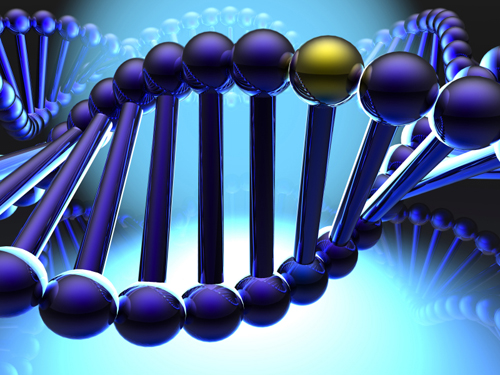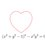# Genetic Algorithm for CryptarithmsAmong passionate puzzle solvers and recreational mathematicians, Cryptarithms are already pretty well-known. A Cryptarithmetic Problem is an equation consisting of words in which letters represent unique digits. To Solve a Cryptarithmetic Problem refers to the process of finiding out the relation between the letters and digits such that the substitution of the letters with their corresponding digits results a mathematically consistent equation.

A famous example of a cryptarithm, due to Henry E. Dudeney, is $\overline{\text{SEND}} + \overline{\text{MORE}} = \overline{\text{MONEY}}$

The solution to this puzzle is

$\left\{ (O \to 0),\\ (M \to 1),\\ (Y \to 2),\\ (E \to 5),\\ (N \to 6),\\ (D \to 7), \\ (R \to 8), \\ (S \to 9) \right\}$

Solution of cryptarithms is an NP-Complete problem.

Though the obvious way to solve the problem would be a depth first search, I found an implementation of Parallel Genetic Algorithms in this paper for solving them:

A. Encoding Individuals

The first part of solving a problem using GA is to define a way to encode the individuals into chromosomes. Assume that we are working with decimal numbers, then we can use an array of characters with length 10 which has indices 0 through 9; whenever we need to assign a digit to a letter we simply put that letter to the cell which has that index of the array. Since each chromosome represents a solution to the problem, changing the place of letters with each other or with empty cells may lead us to a different solution.

B. Fitness Function

Fitness function evaluates the quality of an individual. The fitness function for this problem is defined with the following formula: $FITNESS = |(F+S)-R|$ Assuming R as the result of the operation, F as the first operand and S as the second operand.

When the fitness of individuals gets closer to zero we will get better individuals. If the fitness of an individual is exactly zero then that individual is the correct solution.

C. Mutation

Mutation operator randomly generates two numbers between 0 and 9 and exchanges the content of the cells in these two indices of the chromosome.

The mutation operator should ensure that the exchange is not illegal, which means it should not allow an exchange that would result positioning any start letter of the words in the zero index of the chromosome. Furthermore, a more efficient mutation operator should not allow the exchange of empty cells.

D. Parallelization

I highly recommend you read the paper for the full algorithm. The best thing about it is that it runs much faster than standard DFS.

Here is my python implementation of this algorithm. This implementation is a bit buggy and needs work.4 years, 9 months ago

This discussion board is a place to discuss our Daily Challenges and the math and science related to those challenges. Explanations are more than just a solution — they should explain the steps and thinking strategies that you used to obtain the solution. Comments should further the discussion of math and science.

When posting on Brilliant:

• Use the emojis to react to an explanation, whether you're congratulating a job well done , or just really confused .
• Ask specific questions about the challenge or the steps in somebody's explanation. Well-posed questions can add a lot to the discussion, but posting "I don't understand!" doesn't help anyone.
• Try to contribute something new to the discussion, whether it is an extension, generalization or other idea related to the challenge.

MarkdownAppears as
*italics* or _italics_ italics
**bold** or __bold__ bold
- bulleted- list
• bulleted
• list
1. numbered2. list
1. numbered
2. list
Note: you must add a full line of space before and after lists for them to show up correctly
paragraph 1paragraph 2

paragraph 1

paragraph 2

[example link](https://brilliant.org)example link
> This is a quote
This is a quote
    # I indented these lines
# 4 spaces, and now they show
# up as a code block.

print "hello world"
# I indented these lines
# 4 spaces, and now they show
# up as a code block.

print "hello world"
MathAppears as
Remember to wrap math in $$ ... $$ or $ ... $ to ensure proper formatting.
2 \times 3 $2 \times 3$
2^{34} $2^{34}$
a_{i-1} $a_{i-1}$
\frac{2}{3} $\frac{2}{3}$
\sqrt{2} $\sqrt{2}$
\sum_{i=1}^3 $\sum_{i=1}^3$
\sin \theta $\sin \theta$
\boxed{123} $\boxed{123}$

Sort by:

Paste removed!!

- 4 years, 9 months ago

http://pastebin.com/84tttH4W

Staff - 4 years, 9 months ago